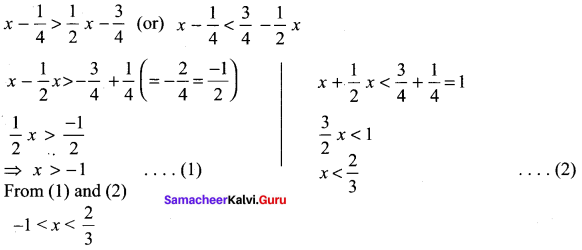## Tamilnadu Samacheer Kalvi 11th Maths Solutions Chapter 2 Basic Algebra Ex 2.2

Question 1.
Solve for x.

(i) |3 – x| < 7
Solution:
⇒ -7 < 3 – x < 7 3 – x > -7
-x > -7 -3 (= -10)
-x > -10 ⇒ x < 10
3 – x < 7
– x < 7 – 3 (= 4)
– x < 4x > -4 … .(2)
From (1) and (2) ⇒ x > -4 and x < 10
⇒ -4 < x < 10

(ii) |4x – 5| ≥ -2
Solution: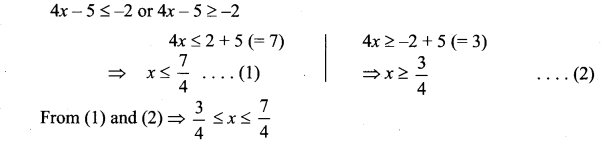(iii)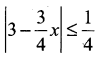Solution: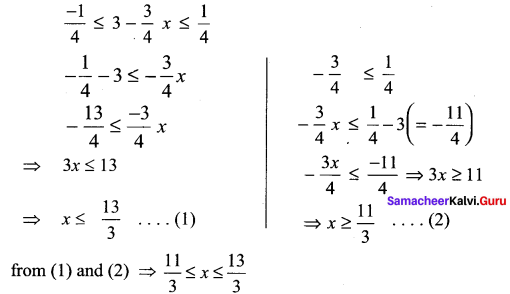(iv) |x| – 10 < -3
Solution:
|x| < -3 + 10 (= 7)
|x| < 7 ⇒ -7 < x < 7Question 2.
Solve $$\frac{1}{|2 x-1|}<6$$ and express the solution using the interval notation.
Solution: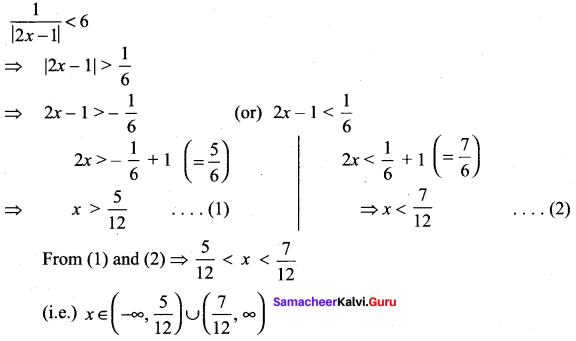Question 3.
Solve -3|x| + 5 ≤ – 2 and graph the solution set in a number line.
Solution:
-3|x| + 5 ≤ – 2
⇒ -3 |x| ≤ – 2 – 5 (= -7)
-3|x| ≤ – 7 ⇒ 3 |x| ≥ 7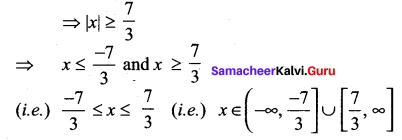Question 4.
Solve 2|x + 1| – 6 ≤ 7 and graph the solution set in a number line.
Solution: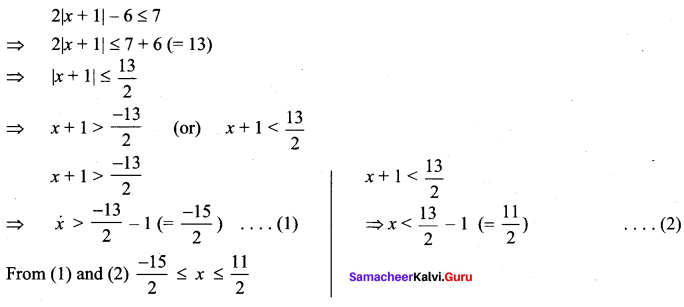Question 5.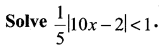Solution: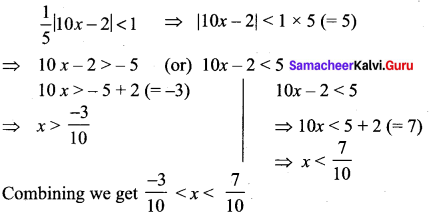Question 6.
Solve |5x – 12| < -2
Solution: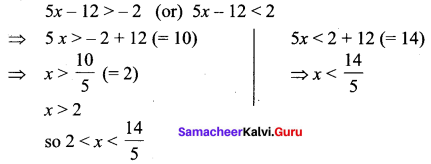### Samacheer Kalvi 11th Maths Solutions Chapter 2 Basic Algebra Ex 2.2 Additional Questions

Question 1.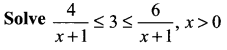Solution:Question 2.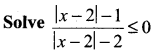Solution: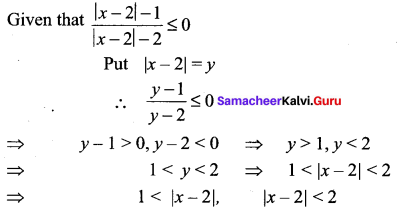⇒ x – 2< – 1 (or) x – 2 > 1 and – 2 < x – 2 < 2
⇒ x < 1 (or) x > 3 and -2 + 2 < x < 2 + 2
⇒ x < 1 (or) x > 3 and 0 < x < 4 Hence, the required solution is (0, 1) ∪ (3, 4)Question 3.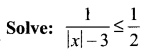Solution: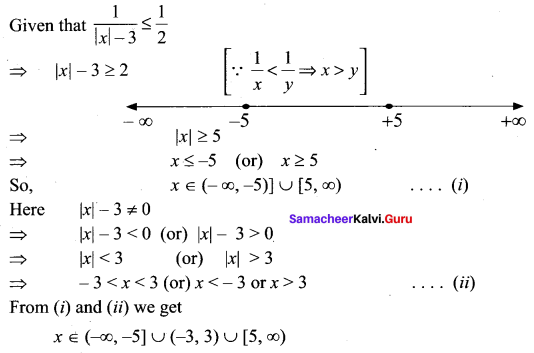Question 4.
Solve: |x – 1| ≤ 5, |x| ≥ 2
Solution:
|x – 1| ≤ 5 and |x| ≥ 2
⇒ -5 ≤ x – 1 ≤ 5 and x ≤ -2 (or) x > 2
⇒ – 5 + 1 ≤ x ≤ 5 + 1
⇒ -4 ≤ x ≤ 6 and x ≤ -2 (or) x ≥ 2
Hence x < [-4, -2] ∪ [2, 6]Question 5.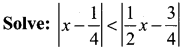Solution: